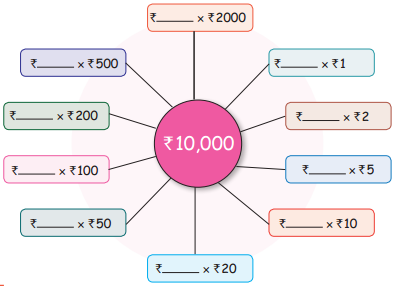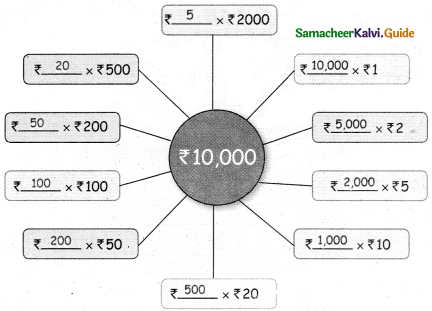Students can download 5th Maths Term 3 Chapter 5 Money InText Questions and Answers, Notes, Samacheer Kalvi 5th Maths Guide Pdf helps you to revise the complete Tamilnadu State Board New Syllabus, helps students complete homework assignments and to score high marks in board exams.

## Tamilnadu Samacheer Kalvi 5th Maths Solutions Term 3 Chapter 5 Money InText Questions

Try This (Text Book Page No.34)

₹ 1 = 100 Paise
₹ 5 = ______ Paise
775 Paise = ₹ 7.75
425 Paise = ₹ ____
₹ 1 = 100 Paise

₹ 5 = ______ Paise
= 500
Hint: ₹ 5 = 5 × 100 paise = 500

775 Paise = ₹ 7.75

425 Paise = ₹ ____
= 4.25
Hint: 425 paise = $$\frac { 425 }{ 100 }$$ = ₹ 4.25Activity (Text Book Page No.37)

Match the big bag with small bag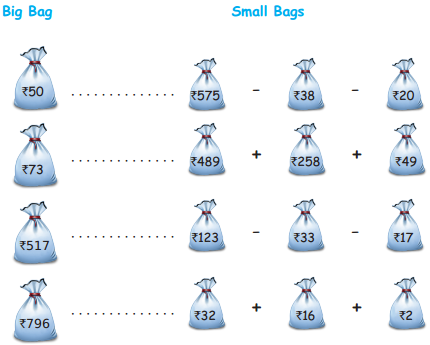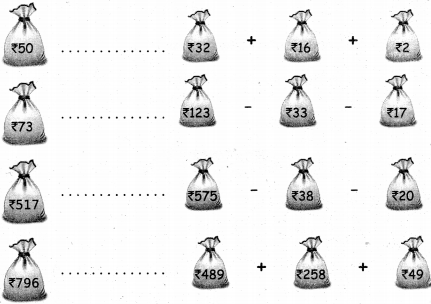Let’s Think (Text Book Page No.41)

Divide 1000 poise equally for 5 students. How many rupees does each student get?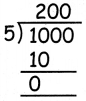200 paise = ₹ $$\frac { 200 }{ 100 }$$ = ₹ 2